# Chapter 2: Numbers and Sequences - Online Test

Q1. Euclid’s division lemma states that for positive integers a and b, there exist unique integers q and r such that a = bq + r , where r must satisfy.
Explaination / Solution:
No Explaination.

Q2. Using Euclid’s division lemma, if the cube of any positive integer is divided by 9 then the possible remainders are
Explaination / Solution: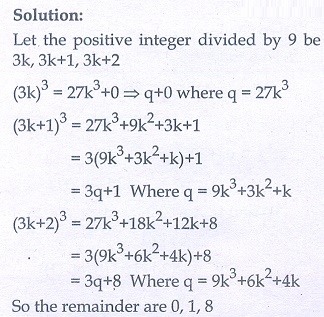Q3. If the HCF of 65 and 117 is expressible in the form of 65m -117 , then the value of m is
Explaination / Solution:Q4. The sum of the exponents of the prime factors in the prime factorization of 1729 is
Explaination / Solution:Q5. The least number that is divisible by all the numbers from 1 to 10 (both inclusive) is
Explaination / Solution: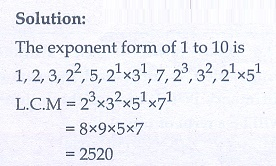Q6. 74k ≡ _____ (mod 100)
Explaination / Solution: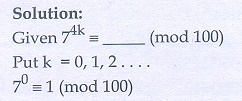Q7. Given F1 = 1 , F2 = 3 and Fn = Fn−1 + Fn−2 then F5 is
Explaination / Solution: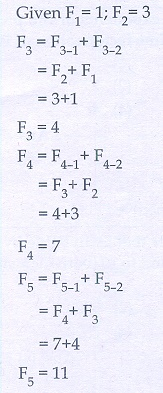Q8. The first term of an arithmetic progression is unity and the common difference is 4. Which of the following will be a term of this A.P.
Explaination / Solution: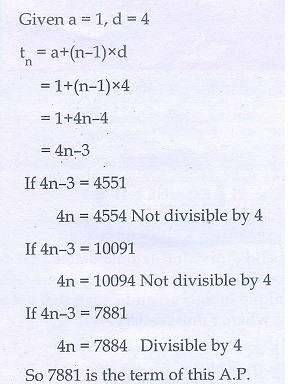Q9. If 6 times of 6th term of an A.P. is equal to 7 times the 7th term, then the 13th term of the A.P. is
Explaination / Solution: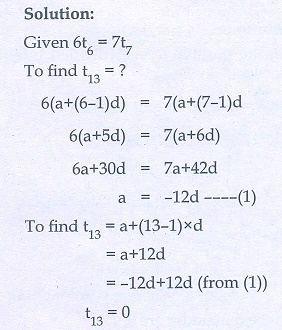Q10. An A.P. consists of 31 terms. If its 16th term is m, then the sum of all the terms of this A.P. is
Explaination / Solution: